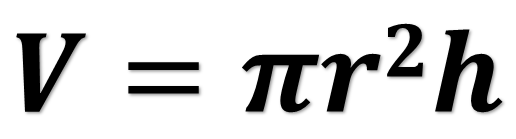#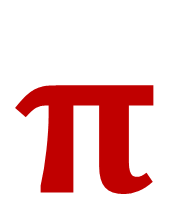DLTK's Crafts for Kids Pi Day Activities - March 14th

Pi is a mathematical number that is the circumference of a circle divided by its diameter. The neat thing about Pi is that when you do that math you get an infinite amount of decimals that never repeat.

Anyways, Pi starts with 3.141592653

3 is also the number for March, then the 14 is the 14th day, the 15 is 2015 , then you can take it further and celebrate at 9:26 in the morning... and add in 53 for 53 seconds.

On March 14, 2015 at 9:26:53am celebrate by eating a piece of PIe!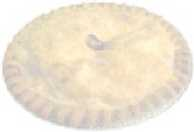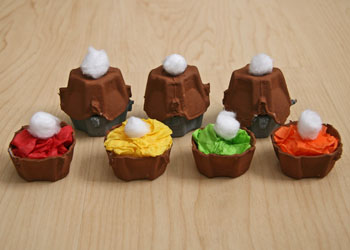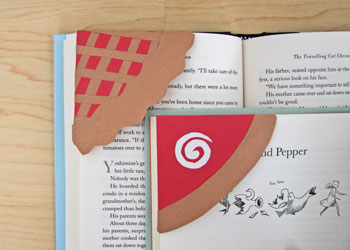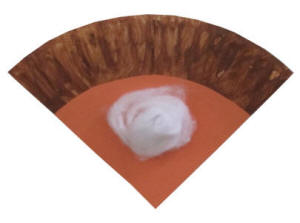Pie Paper Plate Craft - great for fractions practice The one in the photo is pumpkin, but you could paint it red for cherry or blue for blueberry if you prefer!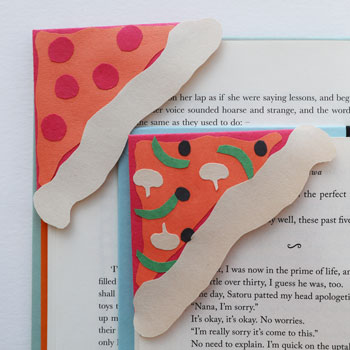For those of you who want to practice some math with pi instead of just eating PIE (or maybe while eating pie?) here are some formulas for you:

### Area of a circle = pi x the radius squared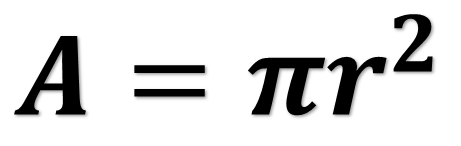### Circumference of a circle = pi x the diameter  (or pi times twice the radius)

(if you imagined a circle as a racetrack, the circumference would be the distance a car would travel if you stayed on the outside edge for one trip around)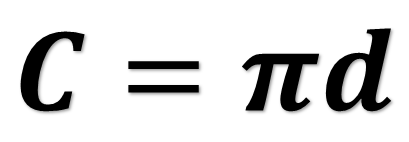or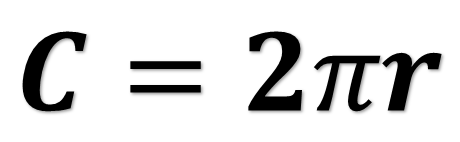### Surface area of a sphere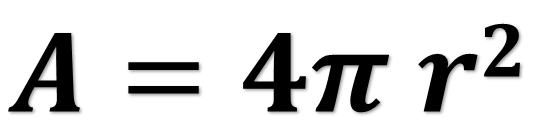### Volume of a cylinder